# Stochastic convergence

Stochastic convergence is a mathematical concept intended to formalize the idea that a sequence of essentially random or unpredictable events sometimes is expected to settle into a pattern.

The pattern may for instance be

• Convergence in the classical sense to a fixed value, perhaps itself coming from a random event
• An increasing similarity of outcomes to what a purely deterministic function would produce
• An increasing preference towards a certain outcome
• An increasing "aversion" against straying far away from a certain outcome

Some less obvious, more theoretical patterns could be

• That the probability distribution describing the next outcome may grow increasingly similar to a certain distribution
• That the series formed by calculating the expected value of the outcome's distance from a particular value may converge to 0
• That the variance of the random variable describing the next event grows smaller and smaller.

## Various possible modes of stochastic convergence

Four different varieties of stochastic convergence are noted:

• Almost sure convergence
• Convergence in probability
• Convergence in distribution
• Convergence in rth order mean

### Almost sure convergence

This is the type of stochastic convergence that is most similar to pointwise convergence known from elementary real analysis.

 Examples of almost sure convergence. Basic example 1 Consider an animal of some short-lived species. We note the exact amount of food that this animal consumes day by day. This sequence of numbers will be unpredictable in advance, but we may be quite certain that one day the number will become zero, and will stay zero forever after. Basic example 2 Consider a man who starts tomorrow to toss seven coins once every morning. Each afternoon, he donates a random amount of money to a certain charity. The first time the result is all tails, however, he will stop permanently. Let X1,X2,... be the day by day amounts the charity receives from him. We may be almost sure that one day this amount will be zero, and stay zero forever after that. However, when we consider any finite number of days, there is a nonzero probability the terminating condition will not occur. Intermediate example A business owner has two sources of income: His business, and interest from a large bank deposit with fixed interest and no withdrawal or deposits. The business income varies unpredictably from month to month, while income from interest is predictable and given by a simple function f. The income for month i can thus be modeled by a random variable Ui = Xi + f(i), where Xi is the income from the business. Now assume Xi converges almost surely to 0 (history bears out that all businesses sooner or later fold up). Then the total monthly income Ui has almost sure convergence to the function f(i).

### Informal description of almost sure convergence

We are confronted with an infinite sequence of random experiments: Experiment 1, experiment 2, experiment 3 ... , where the outcome of each experiment will generate a real number. The random experiments will thus generate a sequence of real numbers, typically denoted x1, x2, x3... .

If we have formulas available that describe the probabilities involved in each experiment, then we may say something about the probability that this sequence will converge to a given value.

If this probability is 1, then the phenomenon of "almost sure convergence" is present.

Note that in advanced treatments the outcomes are not restricted to real numbers.

#### Formal definition

Let X0, X1, X2... be an infinite sequence of random variables defined over a subset of R.

Then the actual outcomes will be an ordinary sequence of real numbers.

If the probability that this sequence will converge to a given real number a equals 1, then we say the original sequence of stochastic variables has almost sure convergence to a.

In more compact notation:

If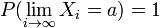$P(\lim_{i \to \infty} X_i = a) = 1$ for some a, then the sequence has almost sure convergence to a.

Note that we may replace the real number a above by a real-valued function f(i) of i, and obtain almost sure convergence to a function rather than a fixed number.

The number a may also be the outcome of a random variable X. In that case the compact but somewhat confusing notation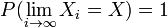$P(\lim_{i \to \infty} X_i = X) = 1$ is often used.

Commonly used notation: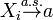$X_i \stackrel{a.s.}{\rightarrow} a$,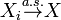$X_i \stackrel{a.s.}{\rightarrow} X$.

### Convergence in probability

The basic idea is that the probability of an "unusual" outcome becomes smaller and smaller as the sequence progresses.

 Illustration of convergence in probability.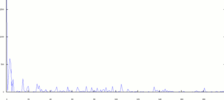Inspecting this graph, you'll notice the behavior typical of convergence in probability.

#### Formal definition

Let$X_0, X_1, ...$ be an infinite sequence of random variables defined over a subset of R.

If there exists a real number a such that$\lim_{i \to \infty} P( |X_i - a| > \varepsilon) = 0$ for all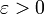$\varepsilon >0$, then the sequence has convergence in probability to a.

Commonly used notation: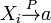$X_i \stackrel{P}{\rightarrow} a$.

### Convergence in distribution

With this mode of convergence, we increasingly expect to see our next outcome in a sequence of random experiments becoming better and better modeled by a given probability distribution.

 Examples of convergence in distribution. Basic example: The outcome from tossing a non-biased dice follows the uniform discrete distribution. Assume a new dice factory has just been built. The first few dices come out quite biased, due to imperfections in the production process. The outcome from tossing any of them will follow a distribution markedly different from the desired uniform discrete distribution. As the factory is improved, the dices will be less and less loaded, and the outcomes from tossing a newly produced dice will follow the desired distribution more and more closely. Intermediate example: Let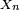$X_n$ be the result of flipping n unbiased coins, and noting the fraction of heads.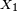$X_1$ will then follow the uniform discrete probability distribution with expected value μ = 0.5 and variance σ2 = 0.25, but as n grows larger,$X_n$ will follow a distribution that gradually takes on more and more similarity to the gaussian distribution . Forming the sequence$Z_n= \frac{ (X_n - \mu) }{\frac {\sigma} {\sqrt {n }}}$, we find the random variables Zn becoming distributed more and more like the standard normal distribution as n increases. We then say the sequence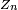$Z_n$ converges in distribution to the standard normal distribution. (This convergence follows from the famous central limit theorem).

#### Formal definition

Given a random variable X with a cumulative distribution function F(x), let Xi be a sequence of random variables, each with cumulative distribution function Fi(x), respectively.

If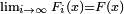$\lim_{i \to \infty} F_i (x) = F(x)$ for all x where F(x) is continuous, then the sequence Xi of stochastic variables converges in distribution to the distribution of X.

Commonly used notation:$X_i \stackrel{L}{\rightarrow} X$. One can also use the distribution directly, so if f.i. X is normally distributed with mean 0 and variance 1, one could write$X_i \stackrel{L}{\rightarrow} N(0,1)$.

### Convergence in r-th order mean

This is a rather "technical" mode of convergence. We essentially compute a sequence of real numbers, one number for each random variable, and check if this sequence is convergent in the ordinary sense.

 Examples of convergence in r-th order mean. Basic example: A newly built factory produces cans of beer. The owners want each can to contain exactly a certain amount. Knowing the details of the current production process, engineers may compute the expected error in a newly produced can. They are continuously improving the production process, so as time goes by, the expected error in a newly produced can tends to zero. This example illustrates convergence in first order mean.

#### Formal definition

If$\lim_{n \to \infty} E(|X_n - a|^r )=0$ for some real number a, then {Xn} converges in r-th order mean to a.

Commonly used notation:$X_n \stackrel{L_r}{\rightarrow} a$.

## Relations between the different modes of convergence

• If a sequence of random variables has almost sure convergence, then it also has convergence in probability.
• If a sequence of random variables has convergence in probability, then it also has convergence in distribution.
• If a sequence of random variables has convergence in (r+1)-th order mean, then it also has convergence in r-th order mean (r>0).
• If a sequence of random variables has convergence in rth order mean, then it also has convergence in probability.